# Mixed fractions calculator

The calculator performs basic and advanced operations with mixed numbers, fractions, integers, decimals. Mixed fractions are also called mixed numbers. A mixed fraction is a whole number and a proper fraction combined, i.e. one and three-quarters. The calculator evaluates the expression or solves the equation with step-by-step calculation progress information. Solve problems with two or more mixed numbers fractions in one expression.

## Result:

### 11/6 = 11/6 = 1 5/6 ≅ 1.8333333

Spelled result in words is one and five sixths (or eleven sixths).

#### Examples:

sum of two mixed numbers: 1 3/4 + 2 3/8
addition of three mixed numbers: 1 3/8 + 6 11/13 + 5 7/8
addition of two mixed numbers: 2 1/2 + 4 2/3
subtracting two mixed numbers: 7 1/2 - 5 3/4
multiplication of mixed numbers: 3 3/4 * 2 2/5
comparing mixed numbers: 3 1/4 2 1/3
changing improper fraction to mixed number: 9/4
What is 3/4 as a mixed number: 3/4
subtracting mixed number and fraction: 1 3/5 - 5/6
sum mixed number and an improper fraction: 1 3/5 + 11/5

## Mixed fractions in word problems:

• Which 5Which mixed number is equivalent to 2.68? A:2 and 6 eighths B:2 and 68 tenths C:2 and 6 over 68
• Mixed2improperWrite the mixed number as an improper fraction. 166 2/3
• A more than bWhat is 5 1/7 more than 2 3/7?
• How much 3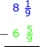How much more is 1⅝ than 4⅚?
• If you 5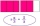If you subtract N from 8 2/5 the difference is 3 4/3. what is N?
• A science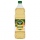A science experiment calls for making 3 and 2/3 cups of distilled water with 1 and 3/5 cups of vinegar and 2/3 cups of liquid detergent. How much liquid in all and cups is needed?
• Mixed to improperChange the given mixed numbers to improper fraction: five-and-four-over-nine (5 4/9)
• Peter's calculationPeter wrote the following: 7 1/4 - 3 3/4 = 4 2/4 = 4 1/2 . Is Peter’s calculation correct? Using words (math vocabulary) and numbers explain why he is correct or incorrect.
• Subtract mixedFind the difference. 5 and two-thirds minus 2 and one-half equals what number? A. three and one-sixth B. three and two-thirds C. three and one-half D. three and five-sixths
• Zailene 2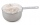Zailene has 8 2/3 cup of flour. She used 5 1/2 cups of flour. How many cups of flour was left to her?
• An electricfan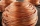An electricfan has a piece of wire is 4 3/8 centimeters long she divides the wire into pieces that are 1 2/3 centimeters long how many pieces does she have?
• Pies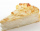Mrs Monica bakes pies. She always cuts each pie into 8 slices. There are 13 slices left on the counter. Write the number of pies on the counter as a mixed number and as an improper fraction.Add this two mixed numbers: 1 5/6 + 2 2/11=Subtract two mixed numbers: 3 1/2 -1 3/4Zailene wants to make two kinds of kakanin, puto and kutsina. Puto recipe needs 3 2/5 cup of flour while Kutsinta needs 5 ¼ cup of flour. How many cups of flour will Zailene be needed in all?What is the difference between 4 2/3 and 3 1/6?Tyron attended his online class 2 hours a day. He already attend 1 3/4 hours, how many more hours will Tyron attend his class?